## Numbers for Letters: the Zip Code Story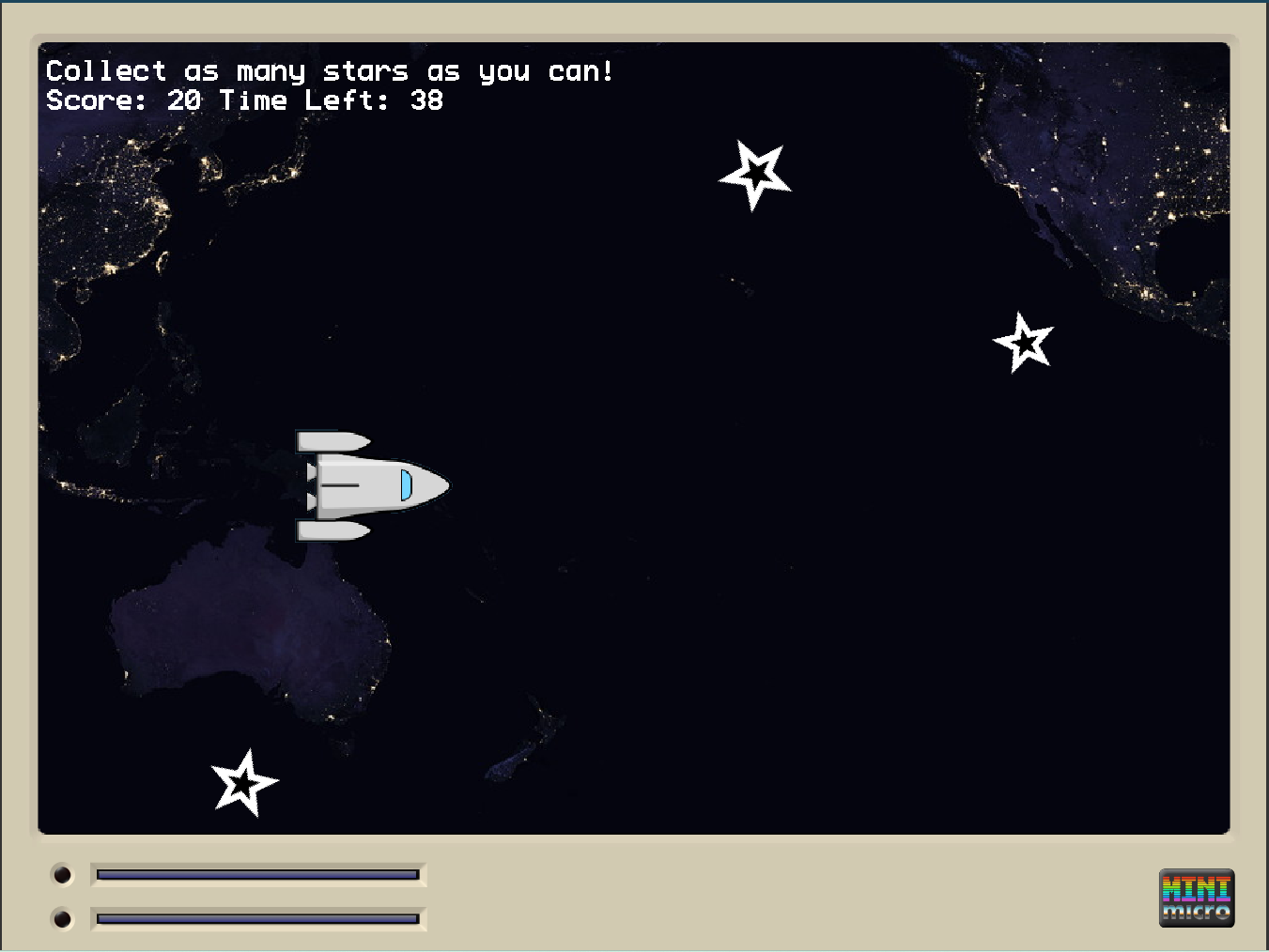## Make a Mini Micro Collection Game## Holy Ozone!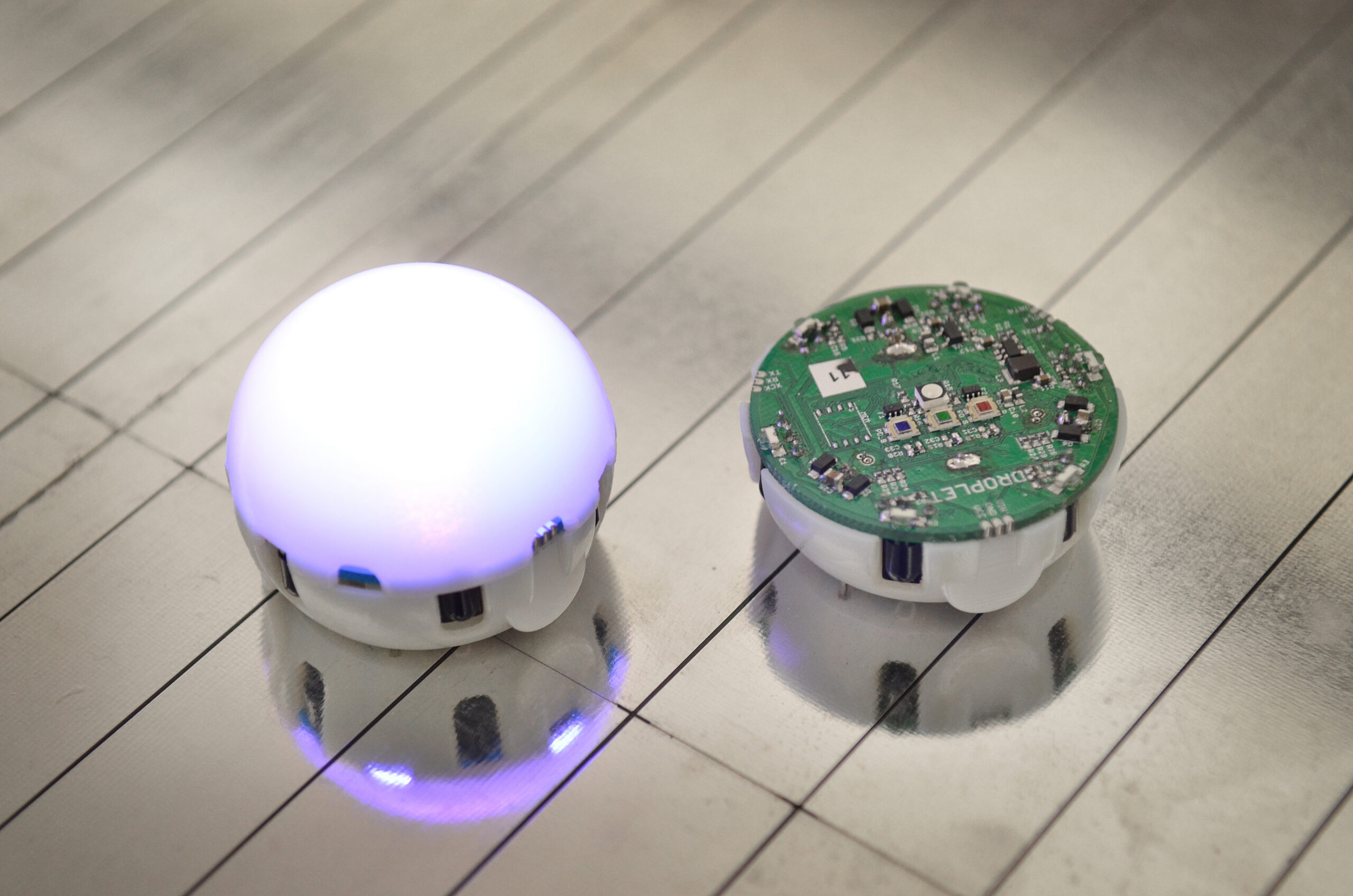#dark mode light mode Search Menu

# Discrete Math: Propositional Logic and Logic Circuits

Matt Hampel on Flickr

How much math do we need to know in order to write computer programs? Perhaps not much. How much math do we need to know to be a computer scientist? It turns out to be quite a bit. What is the difference and why the disparity?

Those who have dabbled in drag and drop languages such as Scratch and Blockly, or even taken a couple courses in a text-based language, may have written many programs without thinking much about mathematics as we learn it in secondary school. The basic skills involve writing a step by step set of instructions that likely includes looping and conditional logic. Looping often doesn’t require much more math than the ability to count, and conditional logic doesn’t resemble much of anything most pre-college students have seen in math class.

Yet, look at the requirements for a college degree in computer science from just about any university and you’re likely to find a class called Discrete Mathematics on the list. What is this class? Why is it required for computer science (but not for almost any other major), and what can we learn about discrete math before college?

As it turns out, just about all middle school, high school, and perhaps even elementary school graduates have done a bit of discrete mathematics. It comes up in the form of basic probability questions such as those involving flipping coins, pulling socks out of drawers, and questions of this sort. However, these types of basic probability questions just scrape the surface of discrete mathematics. The content covered by most discrete math for computer science majors classes is too much to describe in one article, so we’ll start with propositional logic.

Nearly all discrete math classes offered by computer science departments include work in propositional logic. Propositional logic consists of statements that are either true or false (but not both at the same time), and the Boolean operators “and” and “or”. For example, consider the following proposition:

Dinosaurs are extinct and rhinos are not.

This proposition consists of two statements: (1) Dinosaurs are extinct. (2) Rhinos are not extinct.
In order for the whole proposition to be true, both statements must be true. However, rhinos are an endangered species and there is a reasonable chance that by 2031 they will be extinct. If that happens, then at that time the statement will be false. But wait, dinosaurs will still be extinct, so won’t part of the proposition be true and part be false? Yes, but because the operator “and” means that both conditions must be true in order for the entire statement to be true, when rhinos go extinct the proposition will be false.

Try this one: I will go to summer camp or I will stay home.

This time we use the operator “or”. If I go to summer camp the proposition is true. If I stay home the proposition is true. If I do both, the proposition is once again true. The only way to make the proposition false is if I do not go to summer camp and I do not stay home.

What though, does all this have to do with computer science? It turns out that propositional
logic translates into binary numbers, which in turn lead into logic circuits. For example, we can replace each statement in a proposition with a letter for short, and organize the results in a truth table.

In the statement “I will go to summer camp or I will stay home”, we can replace “I will go to summer camp” with the letter “p”, and “I will stay home” with the letter “q”. The “or” operator is represented with the symbol “v”. The result is as follows:

`p v q`

If we want to symbolically list out under what conditions p v q (read “p or q”) is true, we can use the number 1 to indicate true, and the number 0 to indicate false. We start by listing all possible combinations of true and false for statements p and q.

For example, perhaps I go to my grandparents’ house, making both “I will go to camp” false and “I will stay home” false (p ? 0, q ? 0). In this case the entire proposition is false. In another situation however, maybe “I will go to summer camp” is false but “I will stay home” is true (p ? 0, q ? 1). In this case, the original proposition “ I will go to summer camp or I will stay home.” is true. All possible combinations of true and false are listed in the truth table below.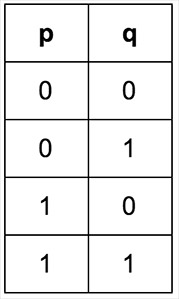We can add a third column to the truth table to evaluate the entire proposition p v q for all four combinations of true and false.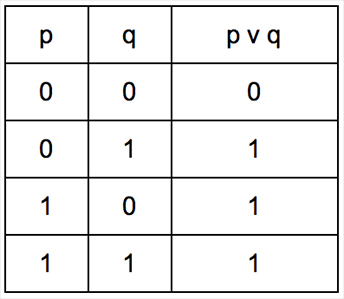If you have ever counted in binary, you might notice a pattern going down the first two columns of the truth table above. We are actually counting from zero to three in binary! If we had a third statement in our proposition, it would translate into counting from zero through seven in binary and so on.

We can even transform the truth table into a logic circuit as shown below. A rounded triangular symbol is used in logic circuits to represent an or gate. Take a look at the simple logic circuit below. There are two inputs, p and q with values of 1 and 0 respectively.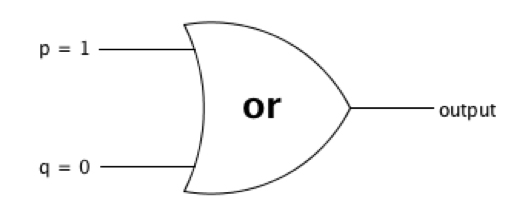Can you guess what the output will be? If you’re not sure, check the truth table for or when p = 1 and a q = 0, or think about the an or statement such as “I will go to summer camp or I will stay home”. In either case, when one part is true and the other is false, the result is true. Therefore, the output of this circuit is 1.

And what about our proposition involving dinosaurs and rhinos? As is, both statements are true and the corresponding logic circuit is shown on the left below. However, if rhinos do indeed become extinct, the logic circuit will change as shown on the right.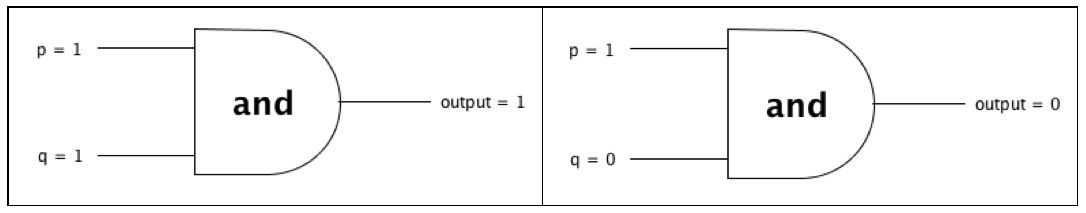By combining multiple “and” and “or” gates, we create increasingly complex logic circuits. The circuit below has a third input. Can you figure out what the output will be?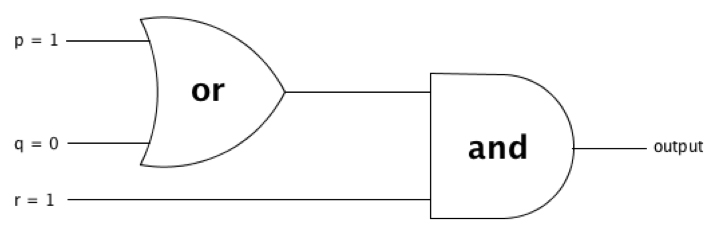If you said the output is 1, give yourself a pat on the back. You are well on your way to mastering a central topic in mathematics for computer scientists!

#### Introduction to Logic

High school material from Stanford
http://logic.stanford.edu/intrologic/secondary/index.html

#### Discrete Mathematics with Applications

https://www.amazon.com/Discrete-Mathematics-Applications-Susanna-Epp/dp/0495391328/ref=sr_1_1?ie=UTF8&qid=1467564041&sr=8-1&keywords=discrete+math+with+applications

#### The Role of Logic in Teaching Proof

http://condor.depaul.edu/sepp/monthly886-899.pdf

#### Language, Proof, and Logic

http://online.stanford.edu/course/language-proof-and-logic-self-paced

##### Related Posts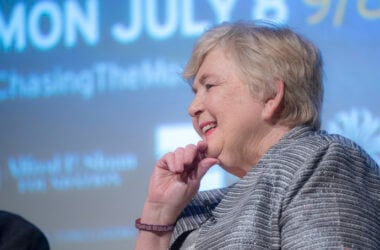## Poppy Northcutt: Getting Apollo to the Moon and Back## Can ChatGPT Code?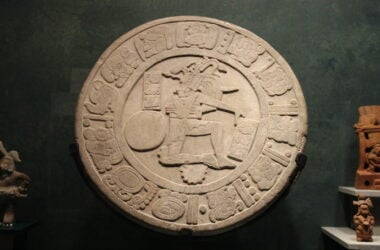## Ancient Mayan Math